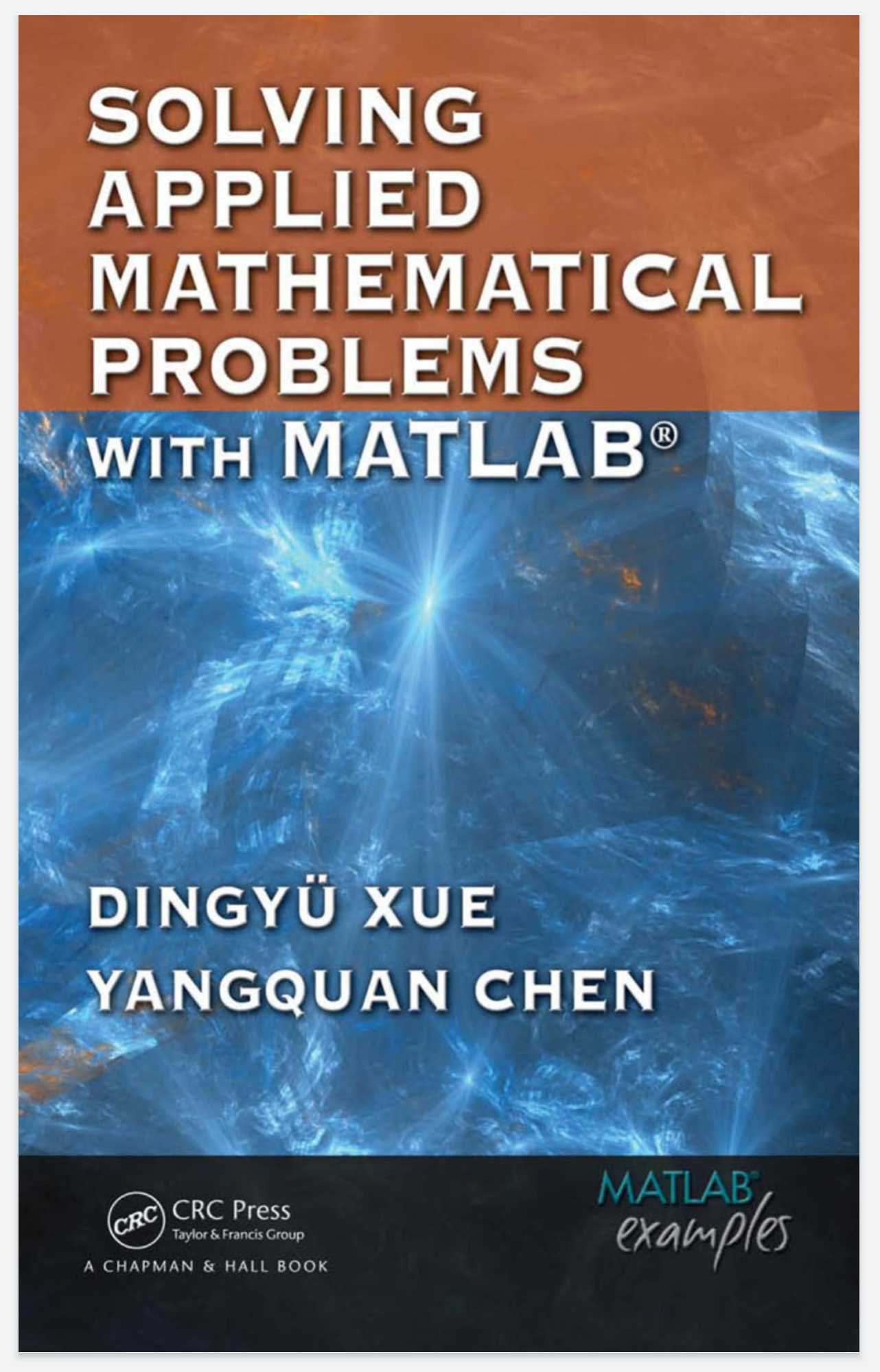Download this PDF book: Solving Applied Mathematical Problems with MATLAB 1st Edition by Dingyu Xue, YangQuan Chen

This textbook presents a variety of applied mathematics topics in science and engineering with an emphasis on problem solving techniques using MATLAB.

The authors provide a general overview of the MATLAB language and its graphics abilities before delving into problem solving, making the book useful for readers without prior MATLAB experience. They explain how to generate code suitable for various applications so that readers can apply the techniques to problems not covered in the book.

Examples, figures, and MATLAB scripts enable readers with basic mathematics knowledge to solve various applied math problems in their fields while avoiding unnecessary technical details.

Computational Thinking, coined and promoted by Jeannette Wing of Carnegie Mellon University, is getting more and more attention. “It represents a universally applicable attitude and skill set everyone, not just computer scientists, would be eager to learn and use” as acknowledged by Dr. Wing, “Computational Thinking draws on math as its foundations.”

The present book responds to “Computational Thinking” by offering the readers enhanced math problem solving ability and therefore, the readers can focus more on “Computational Thinking” instead of “Computational Doing.” The breadth and depth of one’s mathematical knowledge might not match his or her ability to solve mathematical problems.

In today’s applied science and applied engineering, one usually needs to get the mathematical problems at hand solved efficiently in a timely manner without complete understanding of the numerical techniques involved in the solution process. Therefore, today, arguably, it is a trend to focus more on how to formulate the problem in a form suitable for computer solution and on the interpretation of the results generated from the computer.

Publisher ‏ : ‎ Chapman and Hall/CRC; 1st edition (November 3, 2020)

Publication date ‏ : ‎ November 3, 2020

Language ‏ : ‎ English

Pages ‏ : ‎ 446

File : ‎ PDF, 2MB